• 这个 MATLAB 包是下面论文中提出的算法 1 的实现。 S. Basiri，E。Ollila和V. Koivunen，“使用新型功率迭代算法的FastICA的替代推导”，在IEEE信号处理快报中，第1卷。 24，没有。 9，第 1378-1382 页，2017 年 9 ...matlab
• 主要介绍了Java语言实现快速取模算法详解，具有一定参考价值，需要的朋友可以了解下。
• 主要介绍了C语言快速取模算法,包括了算法的分析与改进,是很多程序设计竞赛中常见的算法,需要的朋友可以参考下
• 幂乘算法

2020-08-29 09:30:31
分治算法设计思想 1.逐项相乘时需要做n-1次乘法运算，时间复杂度为o（n）o（n）o（n） 2.当n为偶数时：ana^{n}an=an/2a^{n/2}an/2 * an/2a^{n/2}an/2,an/2a^{n/2}an/2可以只求一次，乘法的运算次数为n/2，运算次数...

问题：求 a n a^{n}

分治算法设计思想

1.逐项相乘时需要做n-1次乘法运算，时间复杂度为 o （ n ） o（n）
2.当n为偶数时： a n a^{n} = a n / 2 a^{n/2} * a n / 2 a^{n/2} , a n / 2 a^{n/2} 可以只求一次，乘法的运算次数为n/2，运算次数减少。当n为奇数时， a n a^{n} = a （ n − 1 ） / 2 a^{（n-1）/2} * a （ n − 1 ） / 2 ∗ a a^{（n-1）/2} * a ，乘法的运算次数为（n-1）/2，同样减少。之后递归求解 a n / 2 a^{n/2} 或者 a （ n − 1 ） / 2 a^{（n-1）/2}
3.时间复杂度分析，规模为n的问题可划分成规模不超过n/2的子问题，
w ( n ) = w ( n / 2 ) + Θ ( 1 ) w(n)=w(n/2)+\Theta(1) 求得 w ( n ) = o ( l o g ( n ) ) w(n)=o(log(n))

代码实现

#include <bits/stdc++.h>
using namespace std;
long long a_n(int a,int n){
long long result;
if(n==1){
result=(long long) a;
return result;
}
if(n%2==0){
result=a_n(a,n/2);
result=result*result;
}
else{
result=a_n(a,(n-1)/2);
result=result*result*a;
}
return result;
}
int main(){
int a=2,n=60;
int result=a_n(a,n);
return 0;
}

算法推广及应用:求矩阵的n次方

代码实现

void  mul_matrix(long long  a[RAW][COL],long long  b[RAW][COL],long long  c[RAW][COL]){
for(int i=0;i<RAW;++i){
for(int k=0;k<COL;++k){
c[i][k]=0;
for(int j=0;j<COL;++j){
c[i][k]+=a[i][j]*b[j][k];
}
}
}
}
void copy_matrix(long long a[][COL],long long b[][COL]){
for(int i=0;i<RAW;++i)
for(int j=0;j<COL;++j)
b[i][j]=a[i][j];
}
void matrix_n(long long a[][COL],long long c[][COL],int n){
if(n==2){
mul_matrix(a,a,c);
return;
}
if(n==1){
copy_matrix(a,c);
return;
}
if(n%2==0){
matrix_n(a,c,n/2);
long long copy_c[RAW][COL];
copy_matrix(c,copy_c);
mul_matrix(copy_c,copy_c,c);
return ;
}
else{
matrix_n(a,c,(n-1)/2);
long long copy_c[RAW][COL];
copy_matrix(c,copy_c);
mul_matrix(copy_c,copy_c,c);
copy_matrix(c,copy_c);
mul_matrix(copy_c,a,c);
}
}

应用：求斐波那契数列

斐波那契数列的性质：
( f ( n + 1 ) f ( n ) f ( n ) f ( n − 1 ) ) \begin{pmatrix}f(n+1) & f(n)\\f(n) &f(n-1)\end{pmatrix} = ( 1 1 1 0 ) n \begin{pmatrix}1 & 1\\1 &0\end{pmatrix} ^ {n}
可归纳证明该性质，再次不赘述，读者可自行百度。
代码实现

int main(){
long long a={1,1,1,0};
long long c={0,0,0,0};
int n=11;//斐波那契数列的第n项
matrix_n(a,c,n);
cout<<c;
return 0;
}

展开全文算法
• 幂等技术及实现方式

千次阅读 2019-07-21 21:36:18
一、什么是幂等 幂等（idempotent）是一个数学与计算机的概念，常见于抽象代数。在编程中一个幂等操作的特点是其任意多次执行所产生的影响均与一次执行的影响相同，也不同担心重复执行会对系统造成改变，例如，...

一、什么是幂等

幂等（idempotent）是一个数学与计算机的概念，常见于抽象代数。在编程中一个幂等操作的特点是其任意多次执行所产生的影响均与一次执行的影响相同，也不同担心重复执行会对系统造成改变，例如，setTrue()函数就是一个幂等函数，无论执行多少次，其结果都是一样的。

二、幂等的实现方案

幂等处理的是多次执行的问题，这并不仅仅出现在并发场景中，无论是顺序执行还是并发执行，都需要做好幂等，而幂等的核心是确保唯一性。实现幂等的方式有很多，比如建立数据库唯一索引、创建唯一数据和状态机约束、乐观锁等。

• 2.1 建立数据库唯一索引

在数据库中创建唯一索引，用作幂等记录，可以防止插入重复数据。以一个插入业务数据的场景为例，可通过业务维度定义唯一索引作为幂等记录，在插入数据方法中，首先查询该幂等记录是否存在，如果存在则直接返回第一次执行的结果，如果不存在则继续执行，并发场景中可能存在多个线程同时插入幂等记录的情况，这种情况下唯一索引可确保只有一个线程可以插入幂等记录成功，其余线程抛异常。插入幂等记录成功的线程可以继续执行后续操作，抛异常的线程执行事务回滚操作。

• 2.2 唯一数据

创建唯一数据的方式有很多种，比如基于tair或redis实现的分布式锁都属于创建唯一数据来实现幂等，以基于tair实现的分布式锁为例:

public boolean tryLock(String lockKey, int expireTime, boolean reentrant) {
...
ResultCode code = tairManager.put(NAMESPACE, lockKey, getLockValue(), DEFAULT_VERSION, expireTime);
...
}

private String getLockValue() {
}

将唯一标识作为key通过tryLock方法，如果返回true，说明当前是第一次调用，继续执行幂等方法，如果返回false，则说明key已经被锁定，这是可选择重试、自旋等待、抛异常等不同策略。

• 2.3 状态机约束

通过状态机的约束可以实现幂等，如果状态机已处于下一个状态，这时候不能往回跳转到上一个状态，通过状态机的跳转约束，可以做到有线状态机的跳转约束，比如基于状态机实现的乐观锁：

update table set status=next_status where id=#{id} and status=#{status}

当前状态第一次被修改后，状态被修改为下一种状态，同一记录针对当前状态的其他修改会失败，程序跑出异常，这常见于并发场景的修改。

• 2.4 基于版本控制的乐观锁

基于版本控制的乐观锁有多种实现方式，比如基于数据库的版本控制乐观锁实现、基于redis的版本控制乐观锁实现等，以数据库的版本控制乐观锁为例：

update table set version=version+1 where id=#{id} and version=#{version}

与基于状态机的乐观锁类似，当前版本第一次本修改后，版本加1，同一记录针对当前版本的其他修改会失败而抛出异常，这常见于并发场景。

参考

展开全文幂等 唯一索引 乐观锁 分布式锁
• 快速幂算法——带你从零开始一步一步优化 目录 快速幂算法——带你从零开始一步一步优化 什么是快速幂算法 再次思考 快速幂算法初步入门 压榨性能再优化 终极优化 参考资料 博客文章版权声明 什么是...

快速幂算法——带你从零开始一步一步优化

目录

快速幂算法——带你从零开始一步一步优化

什么是快速幂算法

再次思考

快速幂算法初步入门

压榨性能再优化

终极优化

参考资料

博客文章版权声明

什么是快速幂算法

首先，我们先来看一道ACM程序设计题，这道题是杭电OJ中序号为2035的题目，没做过这道题目的同学可以跟着一起做一下（点击此处传送），题目如下：

问题描述：这道题目乍一看会觉得并不难啊，题目短短一行而已，而且思路也很容易，求幂这种算法一般在初学程序设计语言的时候应该都有联系过，只要写一个简单的循环就能够搞定。

/**
* 普通的求幂函数
* @param base 底数
* @param power  指数
* @return  求幂结果的最后3位数表示的整数
*/
long long normalPower(long long base,long long power){
long long result=1;
for(int i=1;i<=power;i++){
result=result*base;
}
return result%1000;
}

这道题不是分分钟解决吗？接下来，让我们来写一个主函数测试一下：

int main(){
long long base,power;
cin>>base>>power;

cout<<"base="<<base<<" power="<<power<<" "<<normalPower(base,power)<<endl;

return 0;

}

然后，让我们愉快的来求一下2^100的结果的后三位数表示的整数是什么吧！输出结果如下：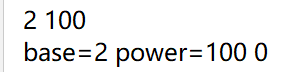为什么答案会是0呢？明明没有错误的啊！~

先不急，我们再来考虑一下，这道题其实出的很有意思，题目要求你输出结果的后三位，为什么不让你直接输出结果呢？难道仅仅只是为了增大题目的难度吗？当然不是，我们在初中就学过“指数爆炸”，下面我们在来回顾一下“指数”的概念：

指数：在乘方a中，其中的a叫做底数，n叫做指数，结果叫幂。

f(x)=a^x ， 随着x单位长度的递增，f(x)会呈“爆炸性”增长。

一张纸对折一次，厚度变成原来的2倍。再对折第二次，变为原来的2的2次方倍即4倍。以此类推，假设纸的厚度为0.1mm，则对折24次以后，长度超过1千米；对折39次达55000千米，超过地球赤道长度；对折42次达44万千米，超过地球至月球的距离；对折51次达22亿千米，超过地球至太阳的距离；对折82次为51113光年，超过银河系半径的长度。

因此，如果题目让你求2的100次方，貌似我们程序设计语言中最大的long lnog类型也无法承载这么大的数值，所以题目才不会要求你输出结果，因为结果可能会非常的大，大到没有任何类型可以承载。所以我们会发现上面的结果为什么是0，因为已经发生溢出了。

那为什么题目要求输出结果的最后三位数表示的整数呢？有的同学可能会问：求一个数的最后三位数表示的整数好办，只要用这个结果进行“取模”运算，让其对1000取模，得到的数就是这个数最后三位数表示的整数。（例如：12345的最后三位数表示的整数是：12345%1000=345）。但是，你这结果都无法求出来，让我怎么进行“取模”运算呢？你这不是瞎闹吗？

别急，我们首先来了解一下“取模”运算的运算法则：（具体的证明感兴趣的同学可以问度娘）

1. (a + b) % p = (a % p + b % p) % p （1）

2. (a - b) % p = (a % p - b % p ) % p （2）

3. (a * b) % p = (a % p * b % p) % p （3）

其中我们只要关注第“3”条法则即可：(a * b) % p = (a % p * b % p) % p ，我们仔细研究一下这个运算法则，会发现多个因子连续的乘积取模的结果等于每个因子取模后的乘积再取模的结果。也就是说，我们如果要求：

(a*b*c)%d=(a%d*b%d*c%d)%d;

因此，我们可以借助这个法则，只需要在循环乘积的每一步都提前进行“取模”运算，而不是等到最后直接对结果“取模”，也能达到同样的效果。

所以，我们的代码可以变成这个样子：

/**
* 普通的求幂函数
* @param base 底数
* @param power  指数
* @return  求幂结果的最后3位数表示的整数
*/
long long normalPower(long long base,long long power){
long long result=1;
for(int i=1;i<=power;i++){
result=result*base;
result=result%1000;
}
return result%1000;
}

我们再来测试一下，这样又能不能输出结果呢？我们仍然来求一下2^100的后三位是什么：这一次完美的得到了我们想要的结果。2^100的幂结果的后三位整数位376。

为了打消一些同学对这个运算法则的怀疑，我们再用一个结果比较小的式子来验证一下：我们知道2^10为1024,按理来说，最后输出的结果的后三位数表示的整数应该是24，那么是不是这样呢？我们来试一试：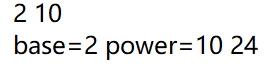最后的结果果然是24，所以这个法则是没有问题的。我们把下面的代码提交给OJ看一下是否能通过：

#include <iostream>
#include <cmath>

using namespace std;

/**
* 普通的求幂函数
* @param base 底数
* @param power  指数
* @return  求幂结果的最后3位数表示的整数
*/
long long normalPower(long long base, long long power) {
long long result = 1;
for (int i = 1; i <= power; i++) {
result = result * base;
result = result % 1000;
}
return result % 1000;
}

int main() {
long long base, power;
while (true) {
cin >> base >> power;
if (base == 0 && power == 0) break;
cout << normalPower(base, power) << endl;
}
return 0;

}

最后的结果是成功Accept了。

再次思考

虽然这个求幂的方法很有用，并且提交给OJ也直接Accept了，但是我们来考虑一下这个算法的时间复杂度，假设我们求2的100次方，那么将会执行100次循环。如果我们分析一下这个算法，就会发现这个算法的时间复杂度为O(N),其中N为指数。求一下小的结果还好，那如果我们要求2的1000000000次方呢？这个程序可能会运行很久很久，具体会多久呢，让我们来测试一下，测试代码如下：

#include <iostream>
#include <cmath>
#include <time.h>

using namespace std;

/**
* 普通的求幂函数
* @param base 底数
* @param power  指数
* @return  求幂结果的最后3位数表示的整数
*/
long long normalPower(long long base, long long power) {
long long result = 1;
for (int i = 1; i <= power; i++) {
result = result * base;
result = result % 1000;
}
return result % 1000;
}

int main() {
clock_t start, finish;
//clock_t为CPU时钟计时单元数
long long base, power;
cin >> base >> power;
start = clock();
//clock()函数返回此时CPU时钟计时单元数
cout << normalPower(base, power) << endl;
finish = clock();
//clock()函数返回此时CPU时钟计时单元数
cout << "the time cost is" << double(finish - start) / CLOCKS_PER_SEC;
//finish与start的差值即为程序运行花费的CPU时钟单元数量，再除每秒CPU有多少个时钟单元，即为程序耗时
return 0;

}

结果如图所示：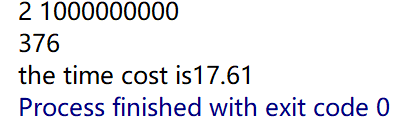我们发现，虽然结果是成功求出来了，但是用了将近18秒的时间才求出最后的答案。这效率当然是非常的低下的，更谈不上实际的生产应用了。那么有没有什么好的办法能够对其进行优化呢？接下来就是我们本次的主题了：快速幂算法。

快速幂算法初步入门

快速幂算法能帮我们算出指数非常大的幂，传统的求幂算法之所以时间复杂度非常高（为O(指数n)），就是因为当指数n非常大的时候，需要执行的循环操作次数也非常大。所以我们快速幂算法的核心思想就是每一步都把指数分成两半，而相应的底数做平方运算。这样不仅能把非常大的指数给不断变小，所需要执行的循环次数也变小，而最后表示的结果却一直不会变。让我们先来看一个简单的例子：

3^10=3*3*3*3*3*3*3*3*3*3

//尽量想办法把指数变小来，这里的指数为10

3^10=(3*3)*(3*3)*(3*3)*(3*3)*(3*3)

3^10=(3*3)^5

3^10=9^5

//此时指数由10缩减一半变成了5，而底数变成了原来的平方，求3^10原本需要执行10次循环操作，求9^5却只需要执行5次循环操作，但是3^10却等于9^5,我们用一次（底数做平方操作）的操作减少了原本一半的循环量，特别是在幂特别大的时候效果非常好，例如2^10000=4^5000,底数只是做了一个小小的平方操作，而指数就从10000变成了5000，减少了5000次的循环操作。

//现在我们的问题是如何把指数5变成原来的一半，5是一个奇数，5的一半是2.5，但是我们知道，指数不能为小数，因此我们不能这么简单粗暴的直接执行5/2，然而，这里还有另一种方法能表示9^5

9^5=（9^4）*（9^1）

//此时我们抽出了一个底数的一次方，这里即为9^1，这个9^1我们先单独移出来,剩下的9^4又能够在执行“缩指数”操作了，把指数缩小一半，底数执行平方操作

9^5=（81^2）*(9^1)

//把指数缩小一半，底数执行平方操作

9^5=（6561^1）*(9^1)

//此时，我们发现指数又变成了一个奇数1，按照上面对指数为奇数的操作方法，应该抽出了一个底数的一次方，这里即为6561^1，这个6561^1我们先单独移出来，但是此时指数却变成了0，也就意味着我们无法再进行“缩指数”操作了。

9^5=（6561^0）*(9^1)*(6561^1)=1*(9^1)*(6561^1)=(9^1)*(6561^1)=9*6561=59049

我们能够发现，最后的结果是9*6561，而9是怎么产生的？是不是当指数为奇数5时，此时底数为9。那6561又是怎么产生的呢？是不是当指数为奇数1时，此时的底数为6561。所以我们能发现一个规律：最后求出的幂结果实际上就是在变化过程中所有当指数为奇数时底数的乘积。

让我们来看一段简单的动画演示（点击放大）：接下来，再让我们用代码来演示一下上面的算法：

long long fastPower(long long base, long long power) {
long long result = 1;
while (power > 0) {
if (power % 2 == 0) {
//如果指数为偶数
power = power / 2;//把指数缩小为一半
base = base * base % 1000;//底数变大成原来的平方
} else {
//如果指数为奇数
power = power - 1;//把指数减去1，使其变成一个偶数
result = result * base % 1000;//此时记得要把指数为奇数时分离出来的底数的一次方收集好
power = power / 2;//此时指数为偶数，可以继续执行操作
base = base * base % 1000;
}
}
return result;
}

我们再来测试一下此时的快速幂算法和普通的求幂算法的效率，我们仍然来求2的1000000000次方，看一看用时又会是多少：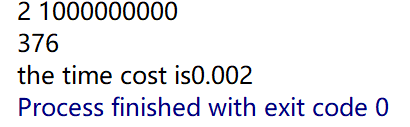真让人简直不可思议，竟然只花了0.002秒就求出了结果，而且结果也是376，然而普通的算法却用了将近18秒的时间才求出最后的结果。

压榨性能再优化

虽然上面的快速幂算法效率已经很高了，但是我们仍然能够再一次的对其进行“压榨级别”的优化。我们上面的代码看起来仍然有些地方可以再进一步地进行简化，例如在if和else代码块中仍然有重复性的代码：

power = power / 2;
base = base * base % 1000;

power = power - 1;//把指数减去1，使其变成一个偶数
power = power / 2;
可以压缩成一句：
power = power / 2;

因为power是一个整数，例如当power是奇数5时,power-1=4,power/2=2；而如果我们直接用power/2=5/2=2。在整型运算中得到的结果是一样的，因此，我们的代码可以压缩成下面这样：

long long fastPower(long long base, long long power) {
long long result = 1;
while (power > 0) {
if (power % 2 == 1) {
result = result * base % 1000;
}
power = power / 2;
base = (base * base) % 1000;
}
return result;
}

接下来，我们来测试一下优化后的性能如何，仍然是求2的1000000000次方：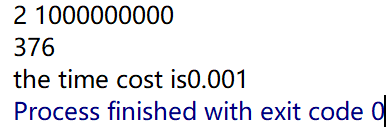结果仍然是正确的376，但时间上的花费从0.002减少成了0.001。

终极优化

在C语言中，power%2==1可以用更快的“位运算”来代替，例如：power&1。因为如果power为偶数，则其二进制表示的最后一位一定是0；如果power是奇数，则其二进制表示的最后一位一定是1。将他们分别与1的二进制做“与”运算，得到的就是power二进制最后一位的数字了，是0则为偶数，是1则为奇数。例如5是奇数，则5&1=1；而6是偶数，则6&1=0；因此奇偶数的判断就可以用“位运算”来替换了。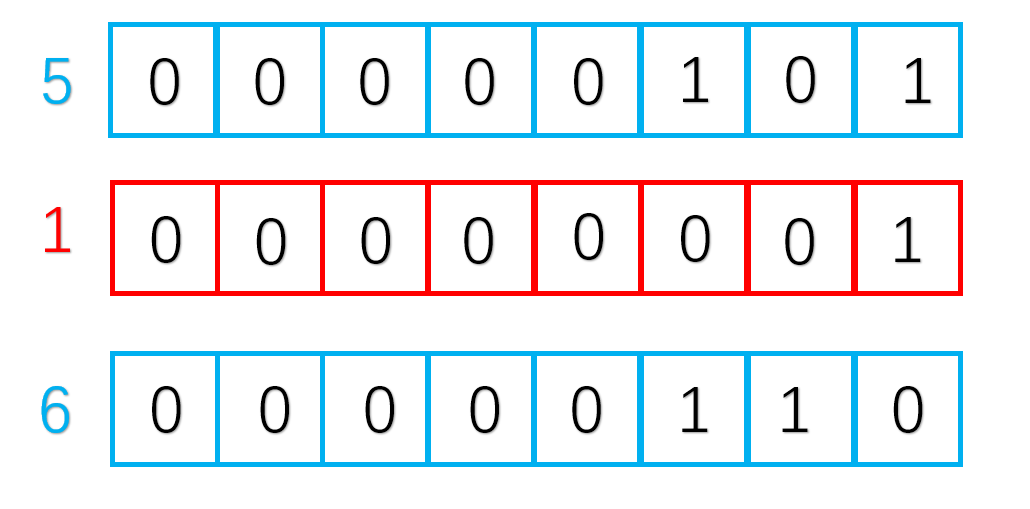同样，对于power=power/2来说，也可以用更快的“位运算”进行替代，我们只要把power的二进制表示向右移动1位就能变成原来的一半了。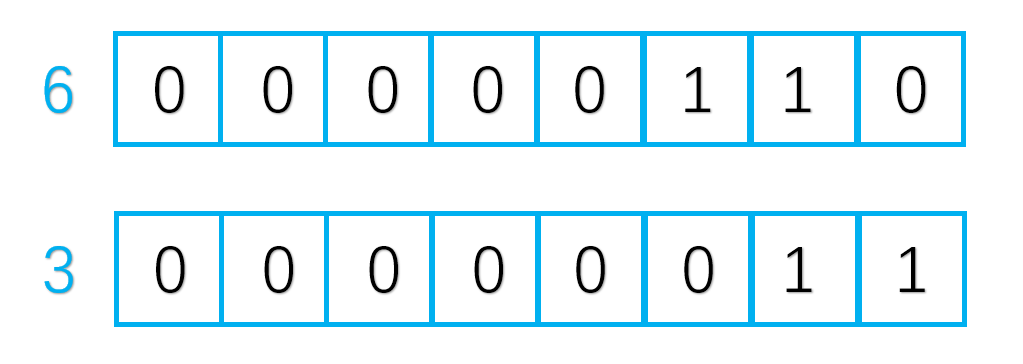最后，我们的代码就能优化成下面这样：
long long fastPower(long long base, long long power) {
long long result = 1;
while (power > 0) {
if (power & 1) {//此处等价于if(power%2==1)
result = result * base % 1000;
}
power >>= 1;//此处等价于power=power/2
base = (base * base) % 1000;
}
return result;
}

我们仍然测试一下求2的1000000000次方，看看终极优化后的代码的性能是怎样的：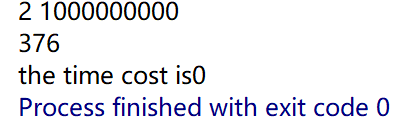简直可怕，时间花费竟然接近于0秒，我们从最开始的18秒最后压缩到接近0秒，真的是感慨算法的威力！如果同样两家公司，采用不同的算法，给用户带来的体验区别是非常大的，这无不让我们感受到算法的威力。

基础不牢？新手不友好？无人带路？关注《扬俊的小屋》公众号吧！参考资料

【2】百度百科——指数爆炸

博客文章版权声明展开全文算法 c++ acm 程序设计
• 使用JS实现表达式求值与运算算法：1）求以a为底的n次递归与非递归实现；2）快速乘法递归与非递归实现；3）表达式求值计算
• 快速幂取模算法详解

千次阅读 2018-05-28 20:24:28
快速取模算法的引入是从大数的小数取模的朴素算法的局限性所提出的，在朴素的方法中我们计算一个数比如5^1003%31是非常消耗我们的计算资源的，在整个计算过程中最麻烦的就是我们的5^1003这个过程 缺点1：在我们在...

1.大数模幂运算的缺陷:
快速幂取模算法的引入是从大数的小数取模的朴素算法的局限性所提出的，在朴素的方法中我们计算一个数比如5^1003%31是非常消耗我们的计算资源的，在整个计算过程中最麻烦的就是我们的5^1003这个过程
缺点1：在我们在之后计算指数的过程中，计算的数字不都拿得增大，非常的占用我们的计算资源（主要是时间，还有空间）
缺点2：我们计算的中间过程数字大的恐怖，我们现有的计算机是没有办法记录这么长的数据的，所以说我们必须要想一个更加高效的方法来解决这个问题
2.快速幂的引入：
我们首先从优化的过程开始一步一步优化我们的模幂算法
2.1.朴素模幂运算过程

#define ans=1
for(int i=1;i<=b;i++)
{
ans*=a;
}

根据我们上面说的，这种算法是非常的无法容忍的，我们在计算的过程中出现的两个缺点在这里都有体现,在这里我们如果要做优化的话，就是在每个过程中都加一次模运算，但是我们首先要记住模运算是非常的消耗内存资源的，在计算的次数非常的大的时候，我们是没有办法忍受这种时间耗费的
2.2.快速幂引入：
在讲解快速幂取模算法之前，我们先将几个必备的知识
2.1.1.对于取模运算：

(a*b)%c=(a%c)*(b%c)%c

这个是成立的：也是我们实现快速幂的基础，之后我们来看看快速幂的核心本质，我通过离散课上的学习，将快速幂的本质差不多理解了一下，感觉还是很深刻的

在这里，我们对指数懂了一些手脚，核心思想在于将大数的幂运算拆解成了相对应的乘法运算，利用上面的式子，始终将我们的运算的数据量控制在c的范围以下，这样我们可以克服朴素的算法的缺点二，我们将计算的数据量压缩了很大一部分，当指数非常大的时候这个优化是更加显著的，我们用Python来做一个实验来看看就知道我们优化的效率有多高了

from time import *
def orginal_algorithm(a,b,c):  #a^b%c
ans=1
a=a%c  #预处理，防止出现a比c大的情况
for i in range(b):
ans=(ans*a)%c
return ans

def quick_algorithm(a,b,c):
a=a%c
ans=1
#这里我们不需要考虑b<0，因为分数没有取模运算
while b!=0:
if b&1:
ans=(ans*a)%c
b>>=1
a=(a*a)%c
return ans

time=clock()
a=eval(input("底数:"))
b=eval(input("指数:"))
c=eval(input("模:"))
print("朴素算法结果%d"%(orginal_algorithm(a,b,c)))
print("朴素算法耗时:%f"%(clock()-time))
time=clock()
print("快速幂算法结果%d"%(quick_algorithm(a,b,c)))
print("快速幂算法耗时:%f"%(clock()-time))

实验结果：

底数:5
指数:1003
模:12
朴素算法结果5
朴素算法耗时:3.289952
快速幂算法结果5
快速幂算法耗时:0.006706

我们现在知道了快速幂取模算法的强大了，我们现在来看核心原理：

对于任何一个整数的模幂运算 a^b%c
对于b我们可以拆成二进制的形式
b=b0+b1*2+b2*2^2+...+bn*2^n
这里我们的b0对应的是b二进制的第一位
那么我们的a^b运算就可以拆解成
a^b0*a^b1*2*...*a^(bn*2^n)
对于b来说，二进制位不是0就是1，那么对于bx为0的项我们的计算结果是1就不用考虑了，我们真正想要的其实是b的非0二进制位

那么假设除去了b的0的二进制位之后我们得到的式子是
a^(bx*2^x)*...*a(bn*2^n)
这里我们再应用我们一开始提到的公式，那么我们的a^b%c运算就可以转化为
(a^(bx*2^x)%c）*...*(a^(bn*2^n)%c)
这样的话，我们就很接近快速幂的本质了，我们会发现令
A1=(a^(bx*2^x)%c)
...
An=(a^(bn*2^n)%c)
这样的话，An始终是A(n-1)的平方倍（当然加进去了取模运算），依次递推

现在，我们基本的内容都已经了解到了，现在我们来考虑实现它

int quick(int a,int b,int c)
{
int ans=1;   //记录结果
a=a%c;   //预处理，使得a处于c的数据范围之下
while(b!=0)
{
if(b&1) ans=(ans*a)%c;   //如果b的二进制位不是0，那么我们的结果是要参与运算的
b>>=1;    //二进制的移位操作，相当于每次除以2，用二进制看，就是我们不断的遍历b的二进制位
a=(a*a)%c;   //不断的加倍
}
return ans;
}

现在，我们的快速幂已经讲完了，我们来大致的推演一下快速幂取模算法的时间复杂度，首先，我们会观察到，我们每次都是将b的规模缩小了2倍，那么很显然，原本的朴素的时间复杂度是O(n)，快速幂的时间复杂度就是O(logn)无限接近常熟的时间复杂度无疑逼朴素的时间复杂度优秀很多，在数据量越大的时候，者中优化效果越明显

快速幂版题

#include"iostream"
#include"cstdio"
#include"cstring"
#include"cstdlib"

using namespace std;

int ans=0;
int a,b;
int c;

int quick(int a,int b,int c)
{
int ans=1;
a=a%c;
while(b!=0)
{
if(b&1) ans=(ans*a)%c;
b>>=1;
a=(a*a)%c;
}
return ans;
}

int main()
{
int for_;
int t;
scanf("%d",&t);
while(t--)
{
ans=0;
scanf("%d%d",&c,&for_);
for(int i=1;i<=for_;i++)
{
scanf("%d%d",&a,&b);
ans=(ans+quick(a,b,c))%c;
}
printf("%d\n",ans);
}
return 0;
}

展开全文•算法
• 幂乘算法及其应用

千次阅读 2018-04-17 20:05:07
不用递归可以直接用循环的形式来模拟算法。#include&lt;stdio.h&gt;int fun(int a,int n){ int r=0; if(n==1) return a; r=fun(a,n/2); if(n%2==0) return r*r; return r*r*a; }int main(){ int a=4;int...
• 快速幂取模快速算法超级详细介绍

万次阅读 多人点赞 2018-04-26 14:59:17
今天在网上看了一些快速取模算法的介绍，总体感觉要么文章介绍的很简略，导致我搞了半天才搞明白什么意思，还有的文章直接放上了错误的代码，真是坑爹啊！所以我就特意写一篇文章方便大家理解一下这个算法的原理和...快速幂取模
• PageRank算法详解 + 示例详解数学求解过程
• 此代码以三维空间为例，编程实现了矩阵特征值的迭代算法。可以扩展到任意维数。
• 为了研究以集合为状态的递推，本文提出了集合级数，并分析了集合级数的性质，总结归纳了三种常见的乘法以及相关快速算法。最后本文给出了几个利用集合级数加速递推的实际例子。算法
• 算法1.首先直接地来设计这个算法： int ans = 1; for(int i = 1;i<=b;i++) { ans = ans * a; } ans = ans % c; 这个算法的时间复杂度体现在for循环中，为O（b）.这个算法存在着明显的问题，如果a和b过大，...
• RSA算法研究，胡云，，根据RSA的算法原理我们可以知道RSA中最主要的运算是执行模运算。BR算法按指数e的二进制重复迭代计算；而 进制算法缩短了序列的长��
• 在RSA密码体制中，加密和解密运算都是模指数运算。计算 可以通过c-1次模乘来实现，然而，如果c非常大，其效率会很低下。 著名的平方-乘可以把计算所需的模乘的次数降低。
• 快速幂取余算法

千次阅读 2015-07-27 20:59:05
先贴一个秦九韶算法（Horner算法）的原理： 设有项的次函数 将前项提取公因子，得 再将括号内的前项提取公因子，得 如此反复提取公因子，最后将函数化为 令 ...算法
• 找自幂数的逐步优化算法

千次阅读 2019-06-14 15:25:07
数是指一个 n 位数，它的每个位上的数字的 n 次之和等于它本身。（例如：当n为3时，有1^3 +...下面分析怎样找出自数的算法： 1：首先要能判断一个自数 (这里面可以分为几个函数 1.找出数字的位数 2.给一个...C语言 优化算法
• C++快速取模代码，可根据需要自行调整优化，刷题的时候计算溢出可以用它解决，算法入门代码，可以直接复制使用
• 分治：幂乘算法

千次阅读 2016-04-30 21:32:07
#include using namespace std; int fz(int a,int n) { if(n==1) return a; if(n%2) return a*fz(a,n/2)*fz(a,n/2); else return fz(a,n/2)*fz(a,n/2); } int main() { int a,n; cin>>a>>n;
• 快速幂算法（数学）

千次阅读 2021-05-21 18:23:26
什么是快速幂算法？快速幂算法能帮我们算出指数非常大的幂，传统的求幂算法之所以时间复杂度非常高(为O(指数n))，就是因为当指数n非常大的时候，需要执行的循环操作次数也非常大。所以我们快速幂算法的核心思想就是...
• 一方面求大数次是一个时间复杂度很高的运算（容易超时），另一方面对1e9+7取模，暗示着结果是连long long都存不下（同余定理），所以这时候快速取模算法就派上用场了，我们先来求a^bmodc; 算法1：直接设计，...
• 快速设计一个高效的求a的n次算法 普通算法 // 时间复杂度O(n) private static int pow0(int a, int n) { int res = 1; for (int i = 0; i < n; i++) { res *= a; } return res; } 改进算法 ...算法
• 主要介绍了C++快速幂算法和大数取模算法的示例，对C++程序员来说有一定的帮助，有需要的朋友可以参考借鉴，下面来一起看看。
•  (1)偶数 a^b mod c = (a^2)^(b/2) mod c(2)奇数 a^b mod c = ((a^2)^(b/2)*a) mod c很明显公式2是可以递推的推论公式:a^b mod c = (a^2 mod c)^(b/2) mod c思路已经比较清晰了,那么具体的算法该如何实现呢......